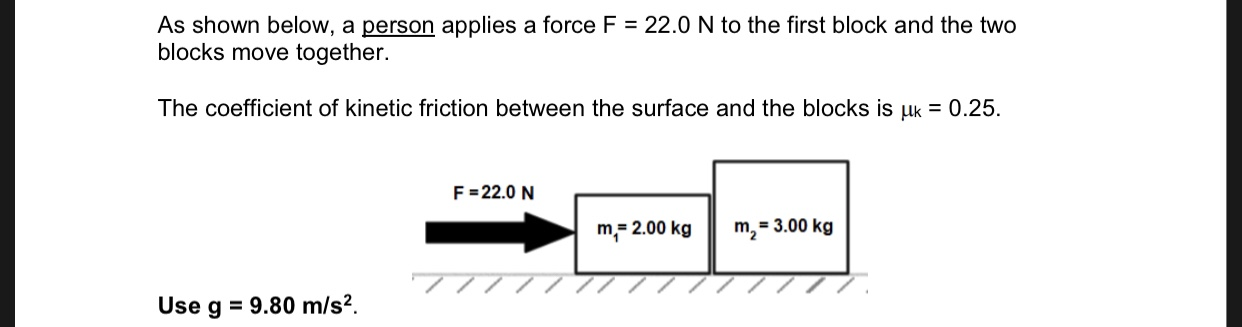# As shown below, a person applies a force F blocks move together. 22.0 N to the first block and the two The coefficient of kinetic friction between the surface and the blocks is uk 0.25 F 22.0 N m2=3.00 kg m 2.00 kg 77 9.80 m/s2. Use g

Question
34 views

a)draw free body diagram for both m1 and m2

b)what is the acceleration of the blocks?help_outlineImage TranscriptioncloseAs shown below, a person applies a force F blocks move together. 22.0 N to the first block and the two The coefficient of kinetic friction between the surface and the blocks is uk 0.25 F 22.0 N m2=3.00 kg m 2.00 kg 77 9.80 m/s2. Use g fullscreen

### Want to see this answer and more?

Step-by-step answers are written by subject experts who are available 24/7. Questions are typically answered within 1 hour.*